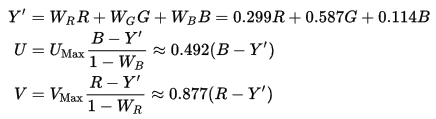# YUV 数据格式详解及python实现

## YUV 数据格式概览

YUV 的原理是把亮度与色度分离，使用 Y、U、V 分别表示亮度，以及蓝色通道与亮度的差值和红色通道与亮度的差值。其中 Y 信号分量除了表示亮度 (luma) 信号外，还含有较多的绿色通道量，单纯的 Y 分量可以显示出完整的黑白图像。U、V 分量分别表示蓝 (blue)、红 (red) 分量信号，它们只含有色彩 (chrominance/color) 信息，所以 YUV 也称为 YCbCr，C 意思可以理解为 (component 或者 color)。Y 中含有三元色色信息，且有较多的 G，所以他们一起可以显示出全彩的图像。## YUV 存储格式

YUV 存储格式有两大类：planar 和 packed。

YUV 码流又根据不同的采样方式分为 YUV4:4:4、YUV4:2:2、YUV4:2:0、YUV4:1:1 等存储格式，其中前 3 种较常见。所谓采样意思就是根据一定的间隔取值。其中的比例是指 Y、U、V 表示的像素，三者分别占的比值。可以按照如下方式理解，实现存储和扫描与 DVD 的扫描线有关。

• YUV4:4:4 是指每个像素分别有一个 Y、一个 U 和一个 V 组成，即每 4 个 Y 采样，就对应 4 个 Cb 和 4 个 Cr 采样，也就是一个像素占用 8+8+8=24 位，这种存储方式图像质量最高，但空间占用也最大，空间占用与 RGB 存储时一样。对于一个 M*N分辨率的图像，该模式下存储空间占用字节数为 M*N*3
• YUV4:2:2 是指每 4 个 Y 采样，对应 2 个 Cb 和 2 个 Cr 采样，这样在解析时就会有一些像素点只有亮度信息而没有色度信息，缺失的色度信息就需要在解析时由相邻的其他色度信息根据一定的算法填充。这种方式下平均一个像素占用空间为 8+4+4=16 位。对于一个 M*N 分辨率的图像，空间占用 16/24，即 M*N*3*(16/24) = M*n*2 个字节。
• YUV4:2:0 是指每 4 个 4 采样，对应 2 个 U 采样或者 2 个 V 采样，注意其中并不是表示 2 个 U 和 0 个 V，而是指无论水平下采样还是垂直下采样，色度采样都只有亮度的一半。该存储格式下，平均每个像素占用空间为 8+4+0=12 位。对于一个 M*N 分辨率的图像来说，空间占用为原来的 12/24，即 M*N*3*(12/24)=M*N*3/2。节省较多存储空间，该存储格式也最常用。
• YUV4:1:1 是指每 4 个 Y 采样，对应 1 个 U 采样和一个 V 采样。平均每个像素占用空间为 8+2+2=12 位。图像空间占用情况同上。这种存储格式实际使用的非常少。

## YV12/I420/YU12/NV12/NV21

YV12/I420/YU12/NV12/NV21 都属于 YUV 4:2:0。YU12 就是 I420，YV12/I420 也称为 YUV420P（即平面格式，planar），YV12 与标准模式 I420 的区别是 UV 顺序不同。
YV12 取名来源是 Y 后面紧跟 V（然后是 U），12 表示它位深为 12，也就是一个像素占用空间为 12 位。

I420: YYYYYYYY UU VV => YUV420P
YV12: YYYYYYYY VV UU => YUV420P
NV12: YYYYYYYY UVUV => YUV420SP
NV21: YYYYYYYY VUVU => YUV420SP

I420 的单帧结构示意图如下（Planar 方式）：NV12 的单帧结构示意图如下（Planar 方式）：## Python的实现：将420P转为jpg

from PIL import Image
def yuv420_to_rgb888(width, height, yuv):
# function requires both width and height to be multiples of 4
if (width % 4) or (height % 4):
raise Exception("width and height must be multiples of 4")
rgb_bytes = bytearray(width*height*3)

red_index = 0
green_index = 1
blue_index = 2
y_index = 0
for row in range(0,height):
u_index = width * height + (row//2)*(width//2)
v_index = u_index + (width*height)//4
for column in range(0,width):
Y = yuv[y_index]
U = yuv[u_index]
V = yuv[v_index]
C = (Y - 16) * 298
D = U - 128
E = V - 128
R = (C + 409*E + 128) // 256
G = (C - 100*D - 208*E + 128) // 256
B = (C + 516 * D + 128) // 256
R = 255 if (R > 255) else (0 if (R < 0) else R)
G = 255 if (G > 255) else (0 if (G < 0) else G)
B = 255 if (B > 255) else (0 if (B < 0) else B)
rgb_bytes[red_index] = R
rgb_bytes[green_index] = G
rgb_bytes[blue_index] = B
u_index += (column % 2)
v_index += (column % 2)
y_index += 1
red_index += 3
green_index += 3
blue_index += 3
return rgb_bytes

def testConversion(source, dest):
print("opening file")
f = open(source, "rb")
f.close()
rgb_bytes = yuv420_to_rgb888(4208,3120, yuv)
# cProfile.runctx('yuv420_to_rgb888(1920,1088, yuv)', {'yuv420_to_rgb888':yuv420_to_rgb888}, {'yuv':yuv})
print("finished conversion. Creating image object")
img = Image.frombytes("RGB", (4208,3120), bytes(rgb_bytes))
print("Image object created. Starting to save")
img.save(dest, "JPEG")
img.close()
print("Save completed")

## Python的实现：将NV21转为jpg

from PIL import Image
def yuv420_to_rgb888(width, height, yuv):
# function requires both width and height to be multiples of 4
if (width % 4) or (height % 4):
raise Exception("width and height must be multiples of 4")
rgb_bytes = bytearray(width*height*3)

red_index = 0
green_index = 1
blue_index = 2
y_index = 0

v_index = width * height

for row in range(0,height):

v_index = width * height + (row//2)*width
u_index = v_index + 1
for column in range(0,width):
Y = yuv[y_index]
#print(y_index)
U = yuv[u_index]
V = yuv[v_index]
C = (Y - 16) * 298
D = U - 128
E = V - 128
R = (C + 409*E + 128) // 256
G = (C - 100*D - 208*E + 128) // 256
B = (C + 516 * D + 128) // 256
R = 255 if (R > 255) else (0 if (R < 0) else R)
G = 255 if (G > 255) else (0 if (G < 0) else G)
B = 255 if (B > 255) else (0 if (B < 0) else B)
rgb_bytes[red_index] = R
rgb_bytes[green_index] = G
rgb_bytes[blue_index] = B
if column==0:
v_index = v_index
elif column%2==0:
v_index = v_index + 2
u_index = v_index + 1
y_index += 1
red_index += 3
green_index += 3
blue_index += 3
return rgb_bytes

def testConversion(source, dest):
print("opening file")
f = open(source, "rb")
f.close()
rgb_bytes = yuv420_to_rgb888(1280,720, yuv)
# cProfile.runctx('yuv420_to_rgb888(1920,1088, yuv)', {'yuv420_to_rgb888':yuv420_to_rgb888}, {'yuv':yuv})
print("finished conversion. Creating image object")
img = Image.frombytes("RGB", (1280,720), bytes(rgb_bytes))
print("Image object created. Starting to save")
img.save(dest, "JPEG")
img.close()
print("Save completed")

testConversion("./test/4.yuv", "4.jpg")©️2019 CSDN 皮肤主题: 大白 设计师: CSDN官方博客